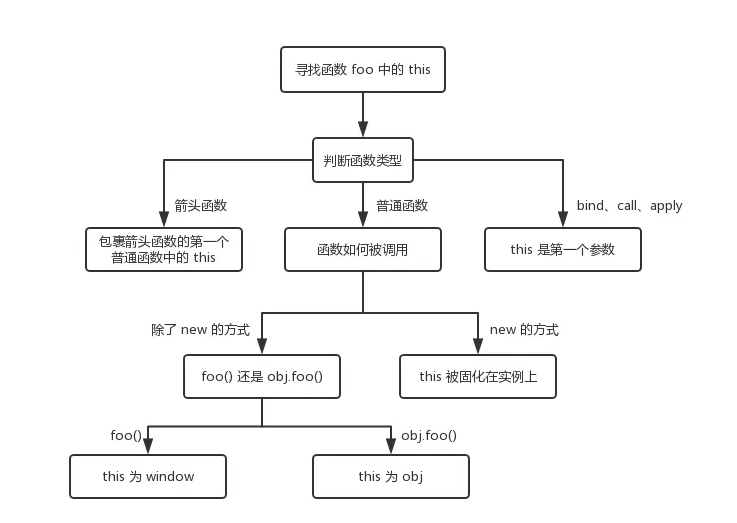# 浏览器中 JavaScript 的执行机制

• 变量提升
• 调用栈
• 作用域链
• 闭包
• this

## 变量提升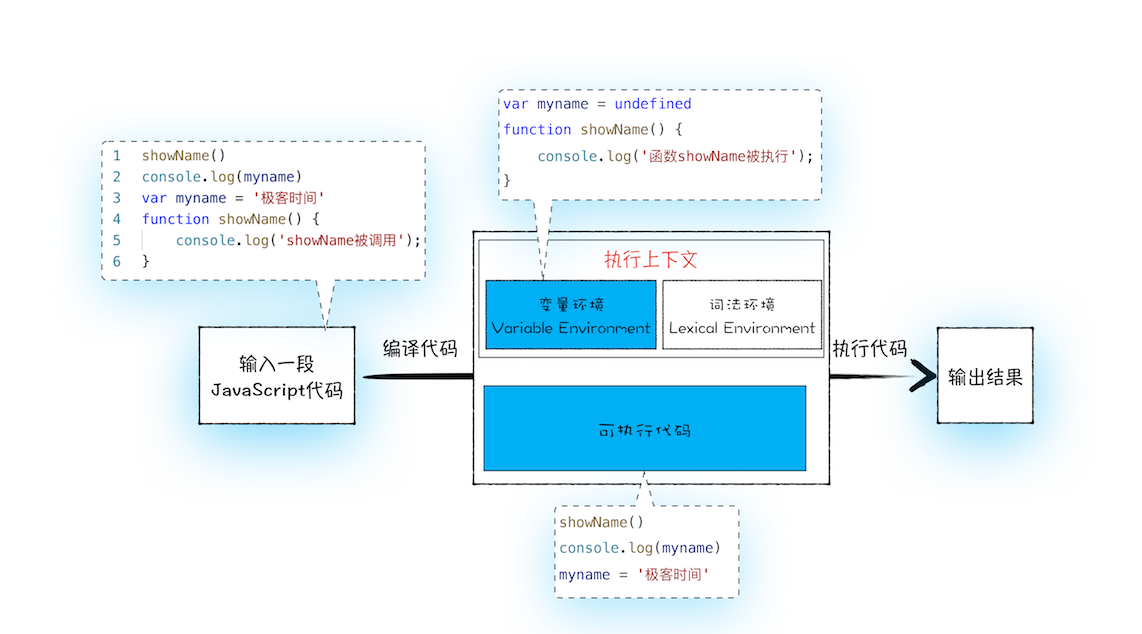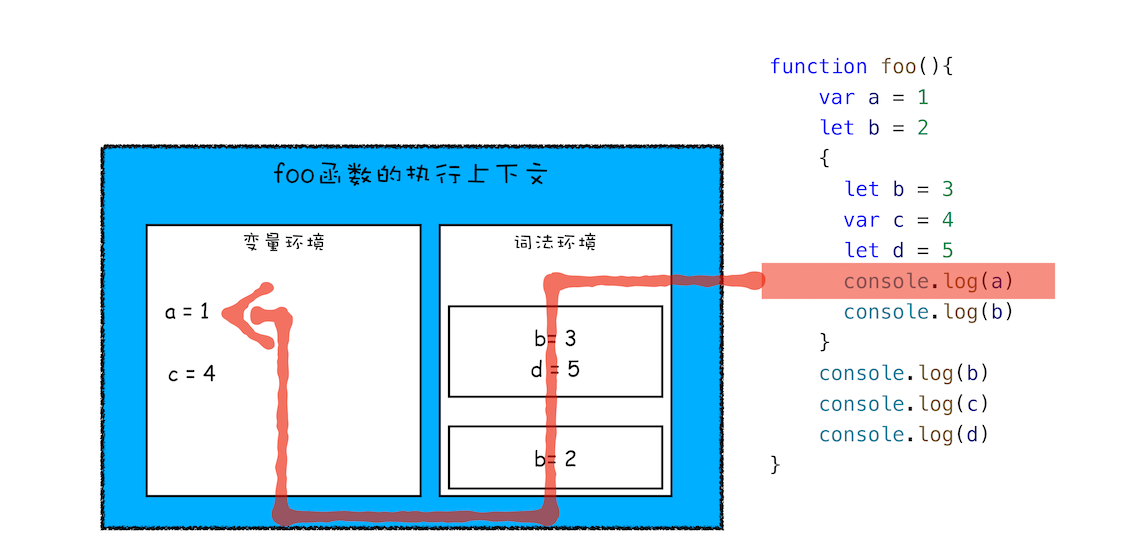• var的创建和初始化被提升，赋值不会被提升。

• let的创建被提升，初始化和赋值不会被提升。

• function的创建、初始化和赋值均会被提升。

## 调用栈

``````var a = 2
return b+c
}
var d = 10
return  a+result+d
}
``````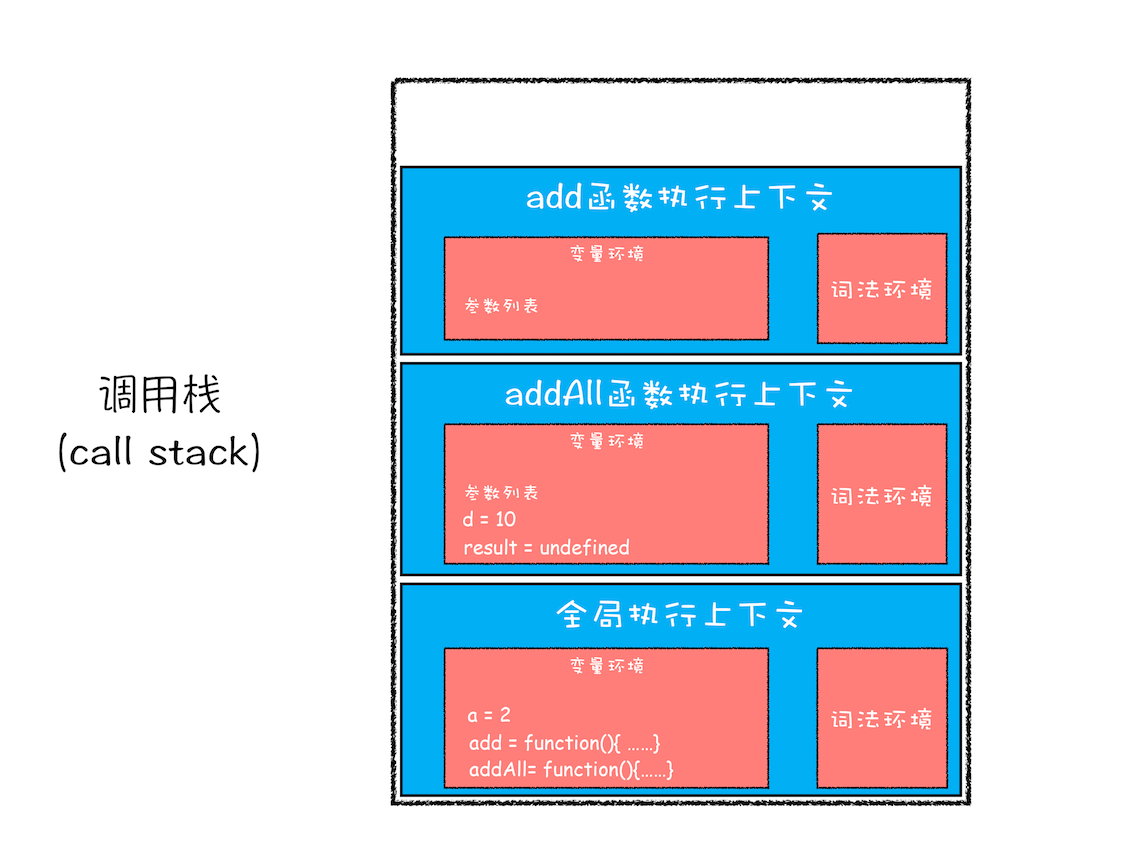``````function Fibonacci2 (n , ac1 = 1 , ac2 = 1) {
if( n <= 1 ) {return ac2};

return Fibonacci2 (n - 1, ac2, ac1 + ac2);
}
Fibonacci2(10000) // Maximum call stack size exceeded
``````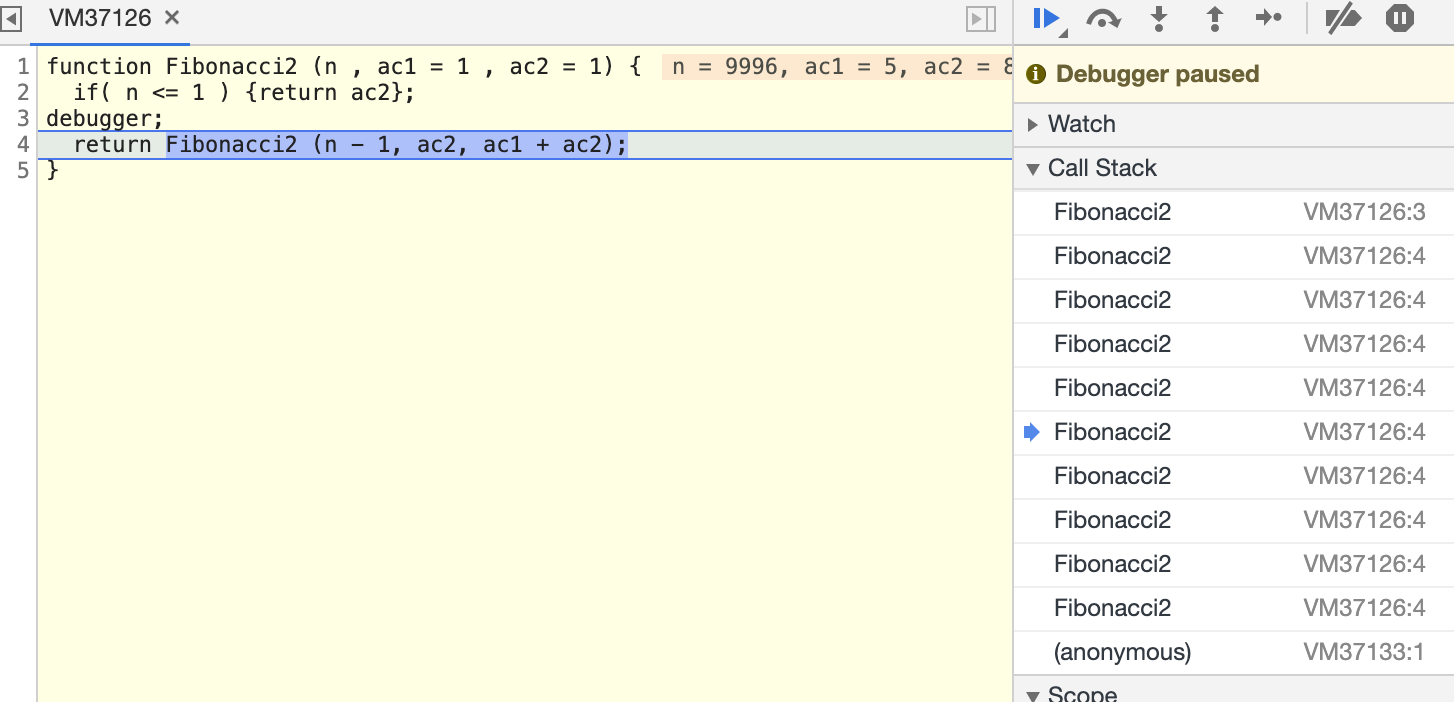• 每调用一个函数，JavaScript 引擎会为其创建执行上下文，并把该执行上下文压入调用栈，然后 JavaScript 引擎开始执行函数代码。
• 如果在一个函数 A 中调用了另外一个函数 B，那么 JavaScript 引擎会为 B 函数创建执行上下文，并将 B 函数的执行上下文压入栈顶。
• 当前函数执行完毕后，JavaScript 引擎会将该函数的执行上下文弹出栈。
• 当分配的调用栈空间被占满时，会引发“堆栈溢出”问题。

``````function runStack (n) {
if (n === 0) return 100;
return runStack.bind(null, n- 2); // 返回自身的一个版本
}
// 蹦床函数，避免递归
function trampoline(f) {
while (f && f instanceof Function) {
f = f();
}
return f;
}
trampoline(runStack(1000000))
``````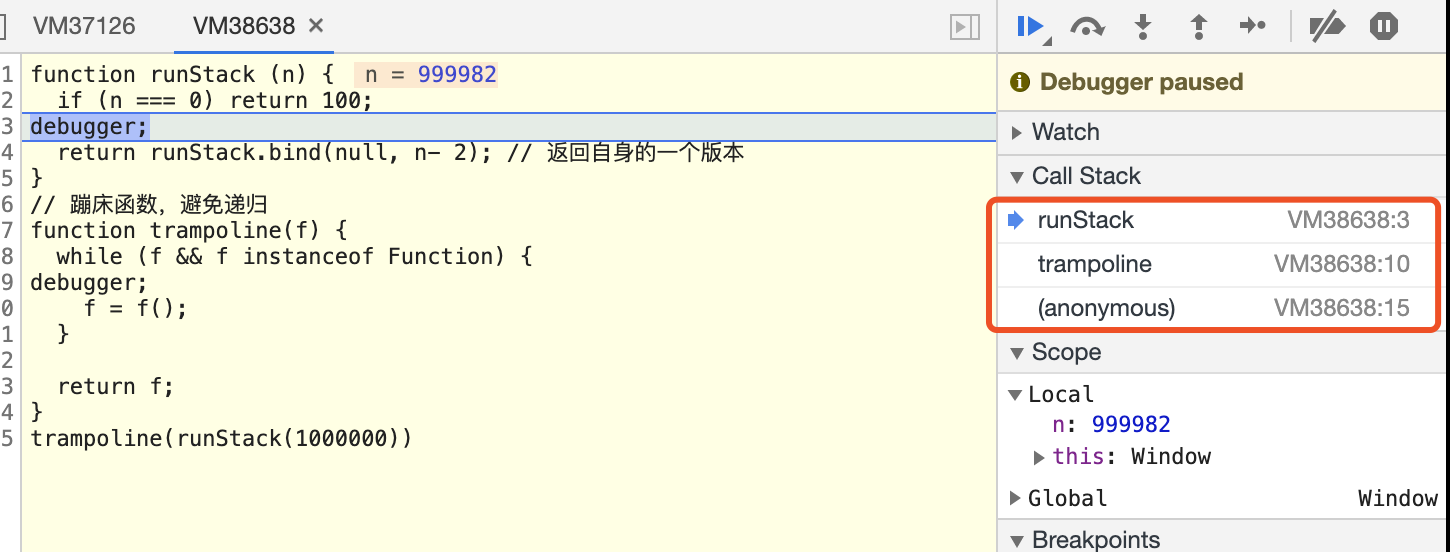## 作用域链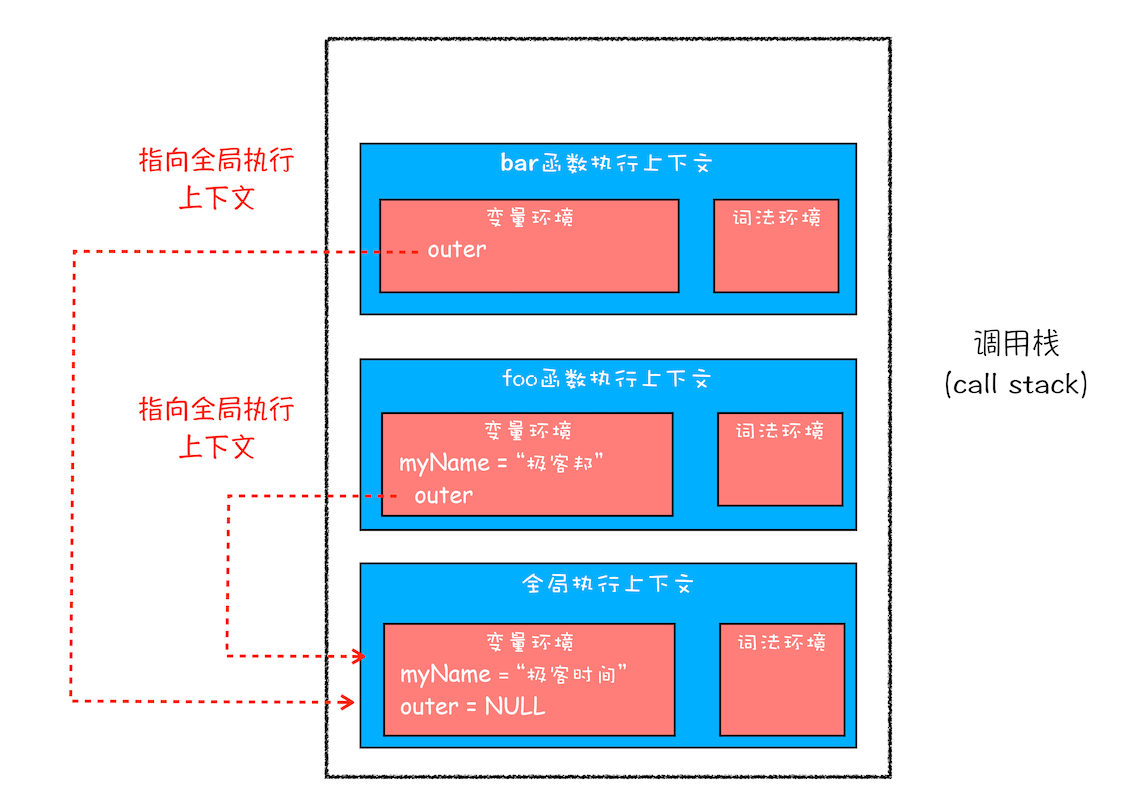### 词法作用域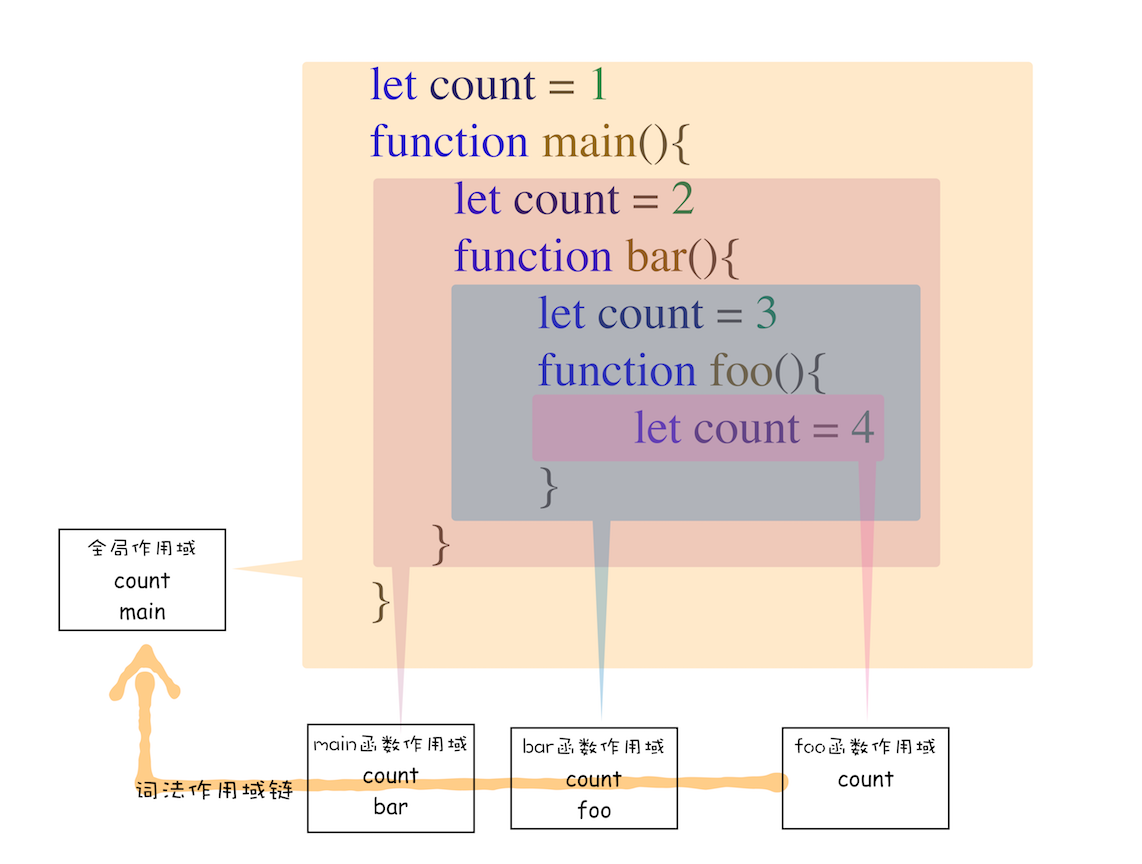### 块级作用域中的变量查找

• 从当前执行上下文的词法环境，自顶向下查找（栈中的内存块），然后再从当前执行向下文中的变量环境中查找；
• 查找不到，则继续在outer指向的执行上下文继续依次先从词法环境，再到变量环境查找。

## this

``````let a = { name: 'this解释' }
function foo() {
console.log(this.name)
}
foo.bind(a)() // => 'this解释''
``````